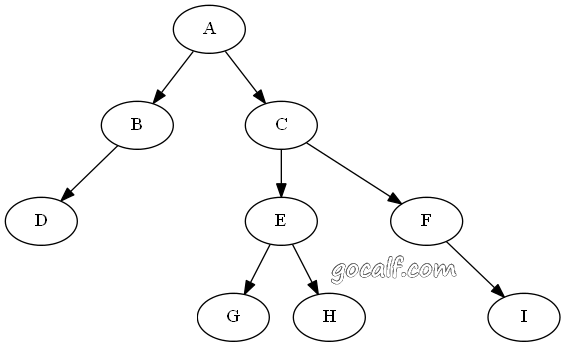• 前序（NLR）：A B D C E G H F I
• 中序（LNR）：D B A G E H C F I
• 后序（LRN）：D B G H E I F C A
• 层序：A B C D E F G H I

## 一、用递归处理二叉树的前序、中序和后序遍历

 ```1 2 3 4 5 6 7``` ```def VisitTree_Recursive(root, order): if root: if order == 'NLR': print(root.data) VisitTree_Recursive(root.left, order) if order == 'LNR': print(root.data) VisitTree_Recursive(root.right, order) if order == 'LRN': print(root.data) ```

## 二、非递归的前序、中序遍历

 ``` 1 2 3 4 5 6 7 8 9 10 11``` ```def VisitTree(root, order): s = [] while root or s: if root: if order == 'NLR': print(root.data) s.append(root) root = root.left else: root = s.pop() if order == 'LNR': print(root.data) root = root.right ```

## 三、非递归的后序遍历

 ``` 1 2 3 4 5 6 7 8 9 10 11 12 13``` ```def VisitTreeLRN(root): s = [] pre = None while root or s: if root: s.append(root) root = root.left elif s[-1].right != pre: root = s[-1].right pre = None else: pre = s.pop() print(pre.data) ```

## 四、非递归的层序遍历

 ``` 1 2 3 4 5 6 7 8 9 10``` ```from collections import deque def VisitTree_LevelOrder(root): if not root: return q = deque([root]) while q: root = q.popleft() print(root.data) if root.left: q.append(root.left) if root.right: q.append(root.right) ```

## 附录

 ``` 1 2 3 4 5 6 7 8 9 10 11 12 13 14 15 16``` ```class Node: def __init__(self, data, left = None, right = None): self.data = data self.left = left self.right = right g = Node('G') h = Node('H') e = Node('E', g, h) i = Node('I') f = Node('F', None, i) c = Node('C', e, f) d = Node('D') b = Node('B', d) a = Node('A', b, c) root = a ```

So what do you think? Did I miss something? Is any part unclear? Leave your comments below.